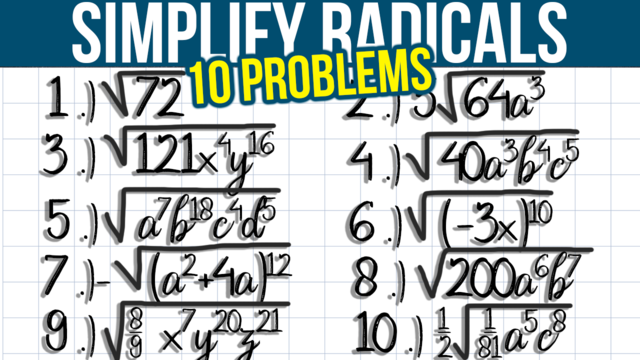# Simplifying Square Roots of Variable Expressions: 10 Examples

Even once you think you understand simplifying square roots of variable expressions, you may still get tripped up by different examples from those you learned in class. I’ll go over examples that we can work through together, step by step, to make sure that we understand what we’re trying to accomplish. If you’re still struggling, please follow along with me. If you think you already have a great idea of how to do them, feel free to work ahead and check your work and answers at the end.

## Example #1: sqrt(72)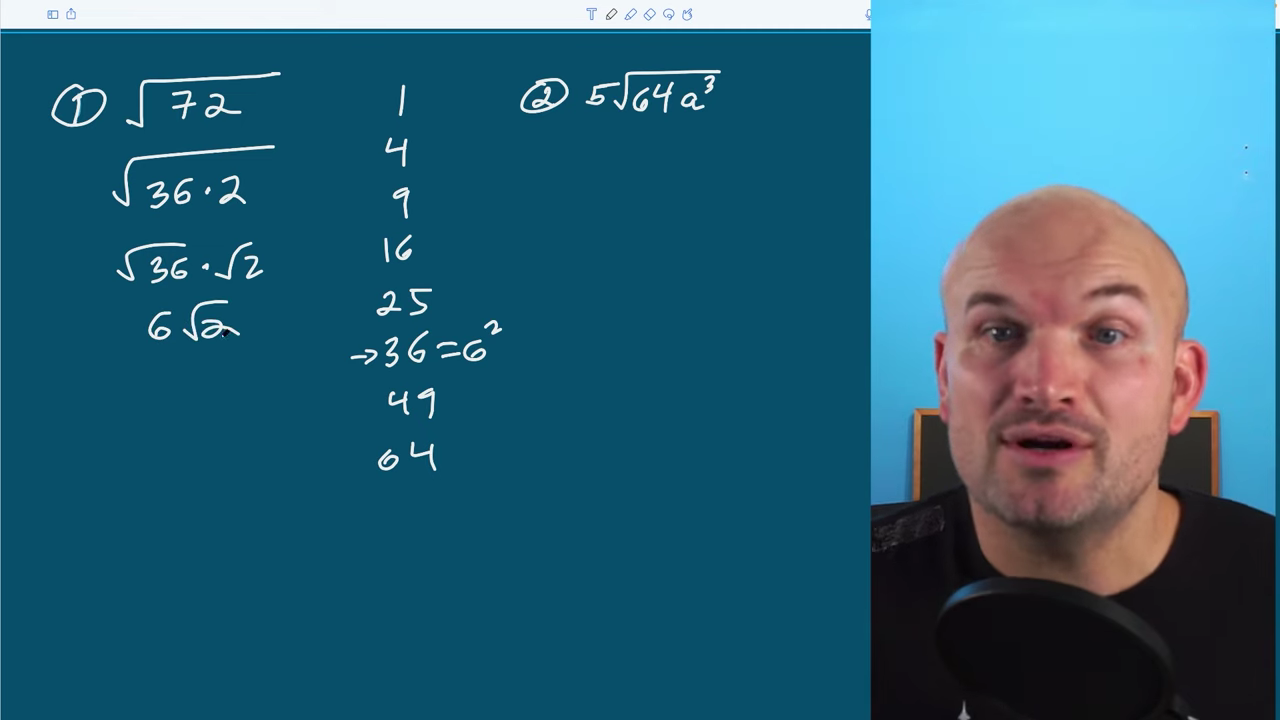The goal of simplifying square root expressions is to rewrite our terms as something squared. We can take the square root of anything raised to the second power, so in this case, I want to find the largest square number that evenly divides into 72 because 72 is not a square number. Out of all numbers that divide into 72, 36 is the highest. 36 is a square number I can rewrite as a 6². I’ll rewrite 72 as √(36⋅2). I can break this up into √36⋅√2. The square root of 36 is 6, making the answer 6√2.

## Example #2: 5sqrt(64a^3)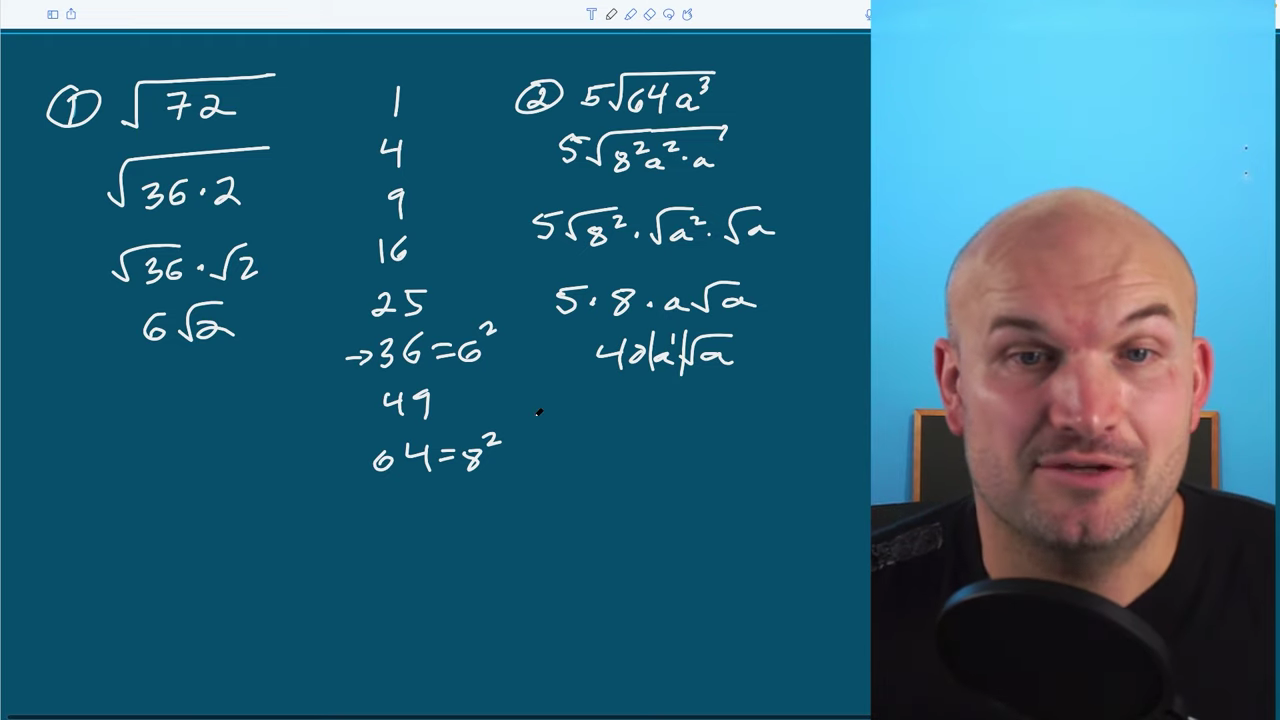64 is a square number that I can rewrite as 8²; a³ is not a square number, but I can rewrite it as a²⋅a. If I break everything up, I’ll get the same expression written as terms raised to the second power. Now, I can break up my terms. Don’t worry about the five on the outside. It’s just a number being multiplied by a radical. We can still break up the radical for each of these terms because they are separated by multiplication.

Now, I can take √8² and √a², which will leave me with 5⋅8⋅a√a, or 40a√a. Since I’m taking the even root and the ending outside of my radical has an odd power, I can also put absolute value to make sure I understand that it will represent a positive value.

## Example #3: sqrt(121x^4y^16)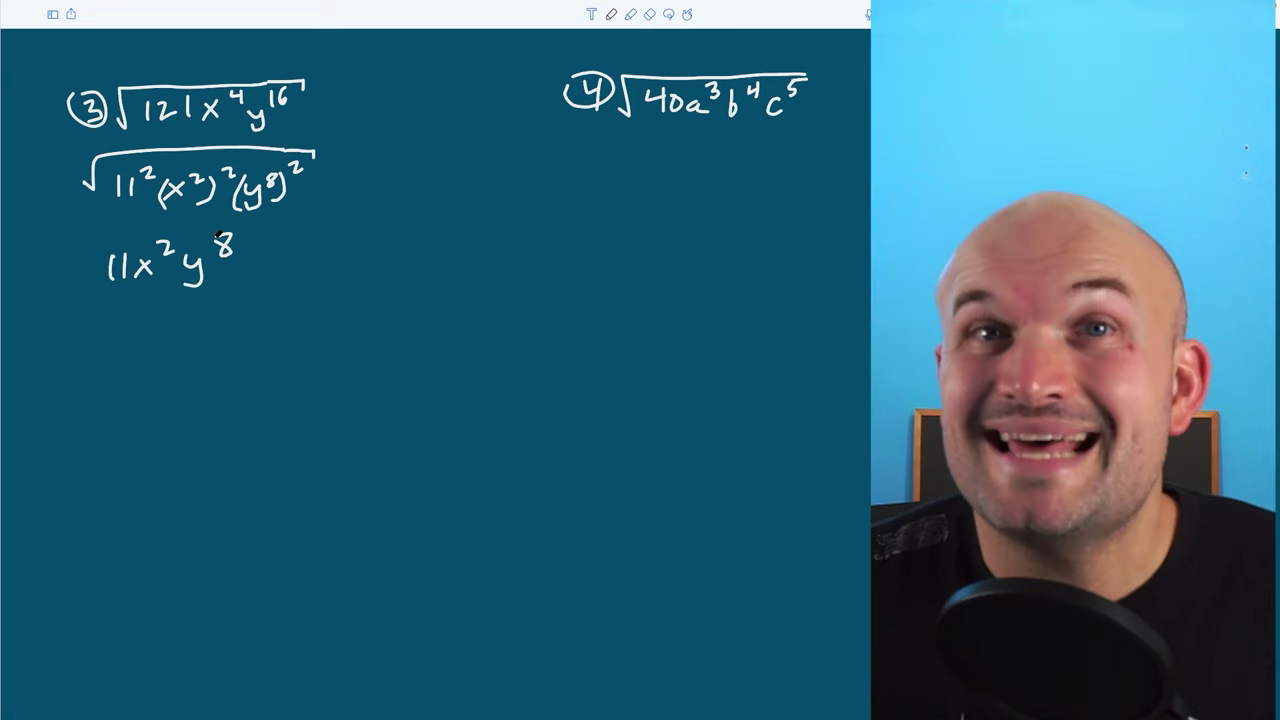The first thing I notice is that 121 is a square number I can rewrite as 11². I could use the product rule for x^4 and write it as x²⋅x², but I don’t want to write y² so many times, so I’ll rewrite it using the power rule. I wrote (y^8)² squared instead of (y²)^8 because I can only take the square root of something being squared. I wrote x^4 as a power rule as well. I could break these up one by one, but you may have noticed that I can take the square root of these separately without writing them and be left with 11x²y^8.

## Example #4: sqrt(40a^3b^4c^5)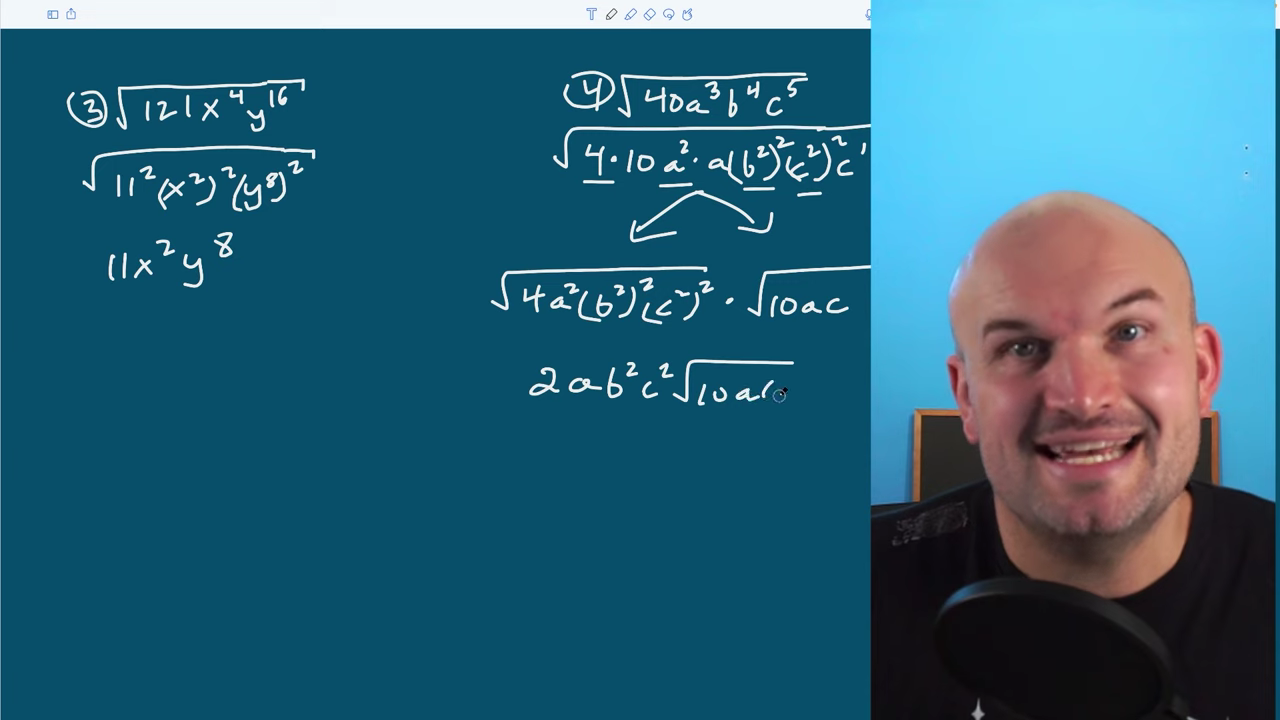In this example, I have three variables. There are many ways to solve this. You can use the product rule or the power rule, but I’ll show you how to use a mix of the two and rewrite this as terms raised to the second power. I’ll rewrite 40 as 4⋅10, a³ as a²⋅a, b^4 as a (b²)², and c^5 as (c²)², which is technically c^4, then multiply by an extra c.

We can also break up our radical. Rather than breaking it up for each term, which can be a lot of work, we can also separate this into terms we can take the square root of and terms we cannot take the square root of. I took the squared terms and wrote 4, a², b², and c². Then, I put them under the radical and multiply them by what’s leftover: 10, a, and c. I can’t simplify any further, but since these are all squared terms, I can now take the square root of them. My final answer is 2ab²c²√10ac.

## Example #5: sqrt(a^7b^18c^4d^5)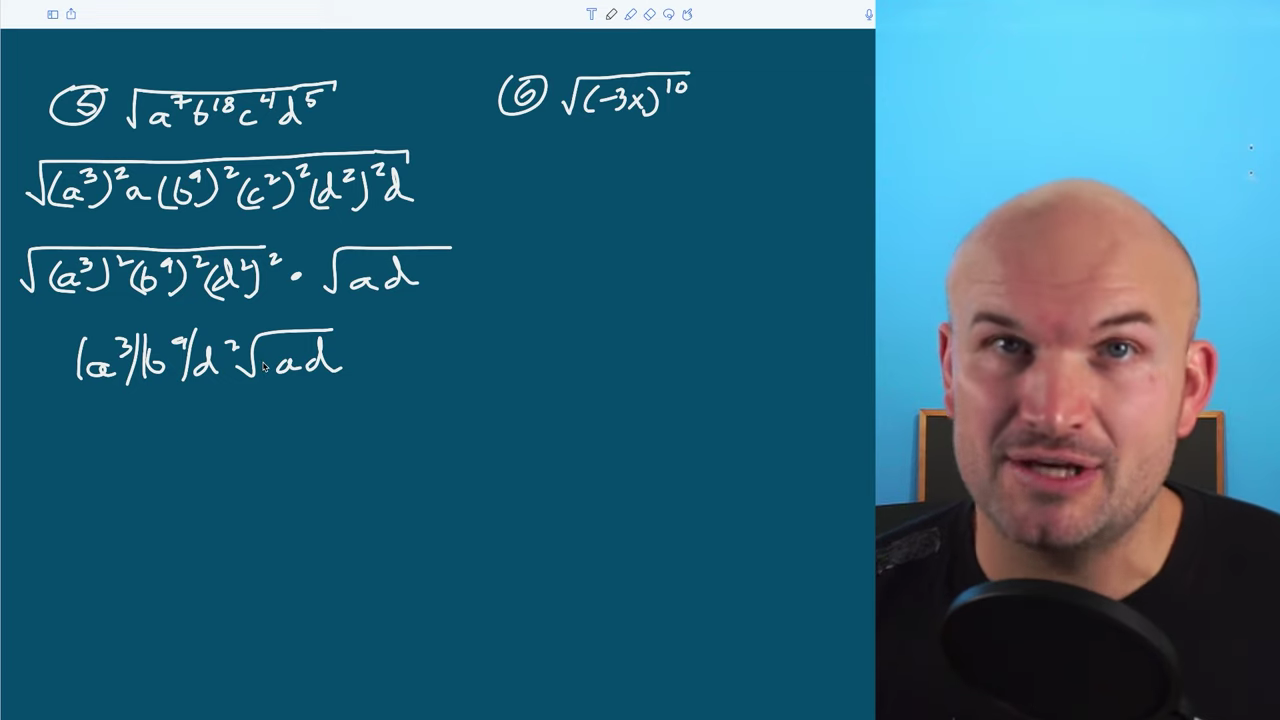Here, we have four variables. I’m going to break them up using a combination of the product rule and the power rule to get to the same powers. If you multiply each term, you’ll get back the original expression. I’ll identify the terms that I can take the square root of and those I cannot. To simplify, I’ll undo the square root and the squaring term, leaving me with a final answer of a³b^9d²√ad. It’s important to recognize that a³ and b^9 are both odd. Since I’m taking the square root and have an odd value, I want to make sure I’m only talking about the positive version. I’m going to use an absolute value. We don’t need to do this for d². Since it’s being squared, it will always be a positive value. If it were negative for the 3rd and the 9th powers, it would give us a negative number we wouldn’t be able to take the square root of. You can’t take the square root of a negative number, so it’s important to be detailed when using absolute values.

## Example #6: sqrt(-3x)^10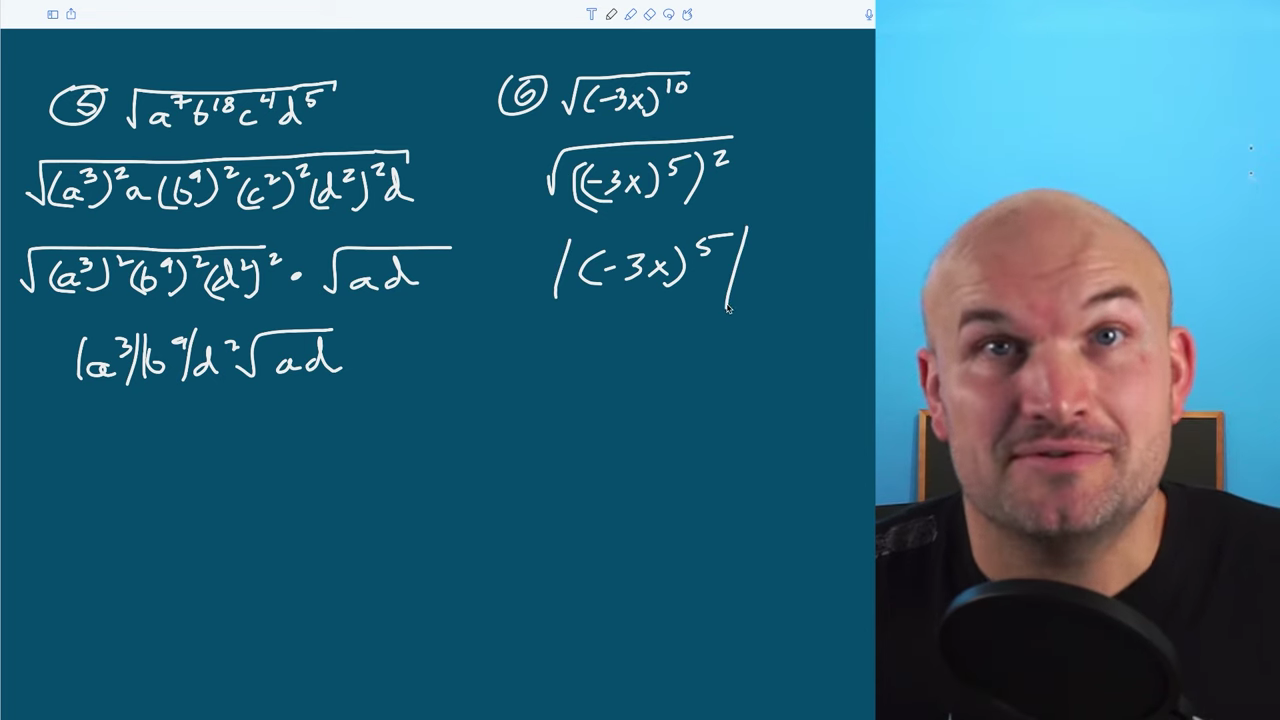In this example, while you can’t take the square root of a negative number, it’s important to understand what you’re doing. (-3x) is under the radical, but it’s being raised to the 10th power. You have to take the square root of the 10th power before you get inside the parentheses, so technically, you’re not taking the square root of a negative number. You’re taking the square root of (-3x)^10. Anything negative raised to an even power will be positive, so all we need to do is rewrite this. I don’t want to rewrite (-3x) ten times, so I’ll rewrite it using the power rule. It’s important to rewrite this as raised to the fifth power squared because I can only take the square root of something squared. They’re inverse operations. My final answer is (-3x)^5, which we know has to be positive because you can’t take the square root of a negative number. We’ll use the absolute value.

## Example #7: sqrt(a^2+4a)^12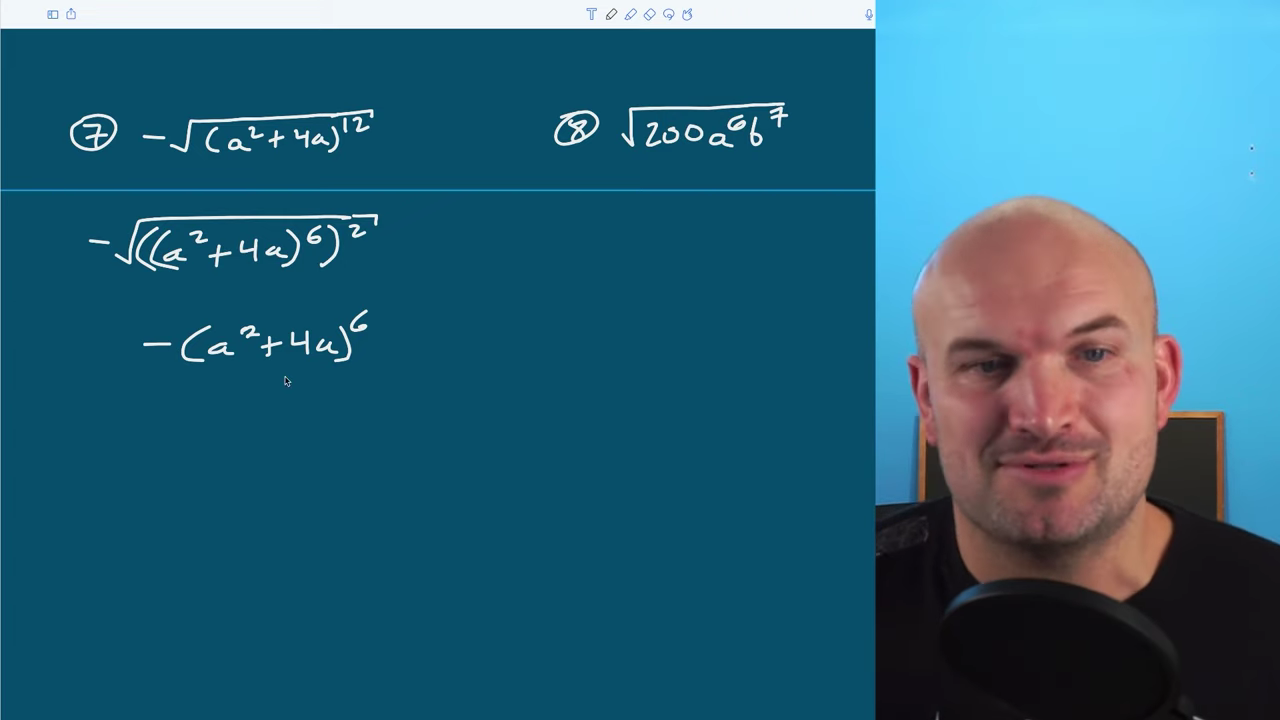This question is very similar to the previous example, though the negative is on the outside. It’s just a scalar; it’s multiplying by your expression. When some people see this expression, they want to distribute the square root to the a² and the 4a because they see those as square numbers. Please do not do this. This expression is raised to the 12th power, so we need to re-simplify that. We can’t distribute the square root across addition and subtraction. I’ll rewrite this expression using the power rule raised to the second power. Now, I can take the square root of that square, leaving me with a final answer of -(a² + 4a)^6. Since it’s even, we don’t need to include the absolute value because this negative is just multiplying the final answer.

## Example #8: sqrt(200a^6b^7)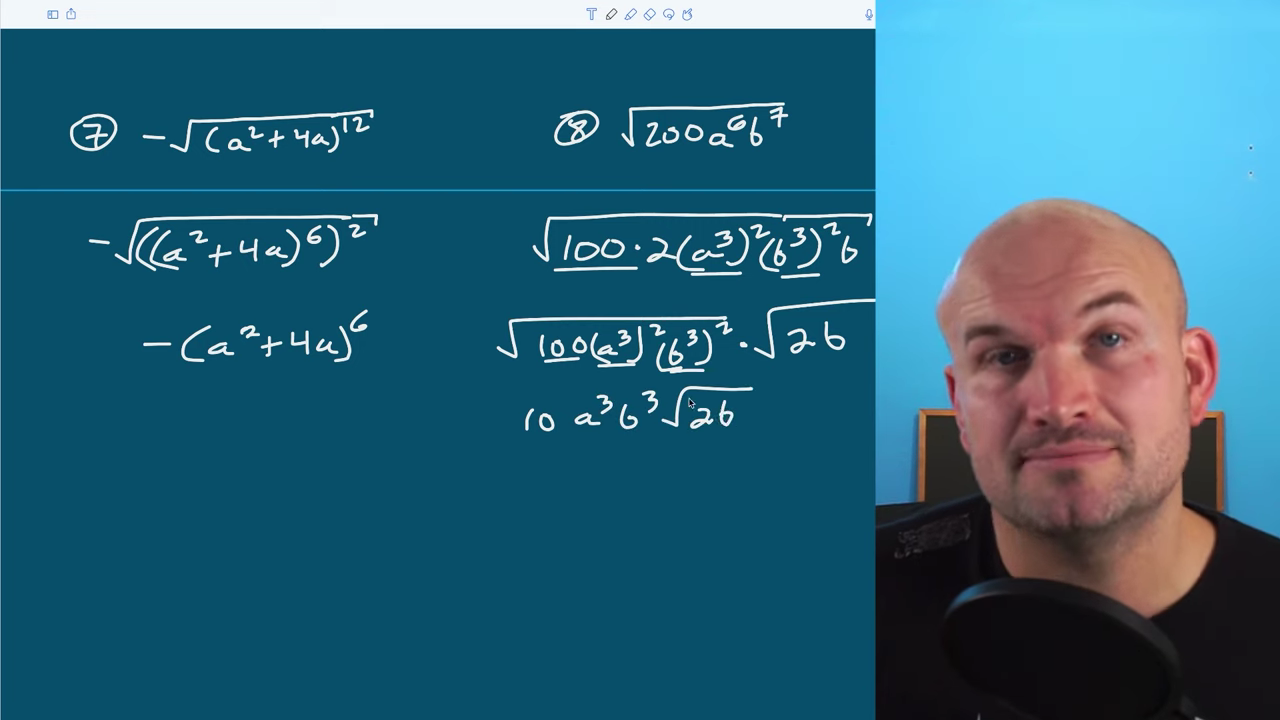We can break this up into 100⋅2 and rewrite it using the power and product rules. Now, I can take the square root of all of my square terms and get 100(a³)²(b³)². I’ll rewrite those under their own radical and the ones I cannot simplify under the other radical. Now, I can take the square root of each one of these terms. You could break them up separately or leave it as 10a³b³√2b.

## Example #9: sqrt(8/9x^7y^20z^21)

Don’t overthink when you see fractions under the radical because just like we can separate our radicand across multiplication, we can also separate it across division. First, I’ll separate by multiplication and then by division. Once I’ve done that, I’ll simplify. I can rewrite √8 as 4⋅2 and break everything up. I can rewrite 9 as √3² because it’s a square number. I’ll break up my variables using the power and the product rules, ensuring I have them raised to the second power. I’ll use the product rule for anything leftover. I broke up 8 as 4⋅2, 9 as 3², x^7 as (x³)²x, y^20 as (y^10)², and z^21 as (z^10)²⋅z to get it to the 21st power.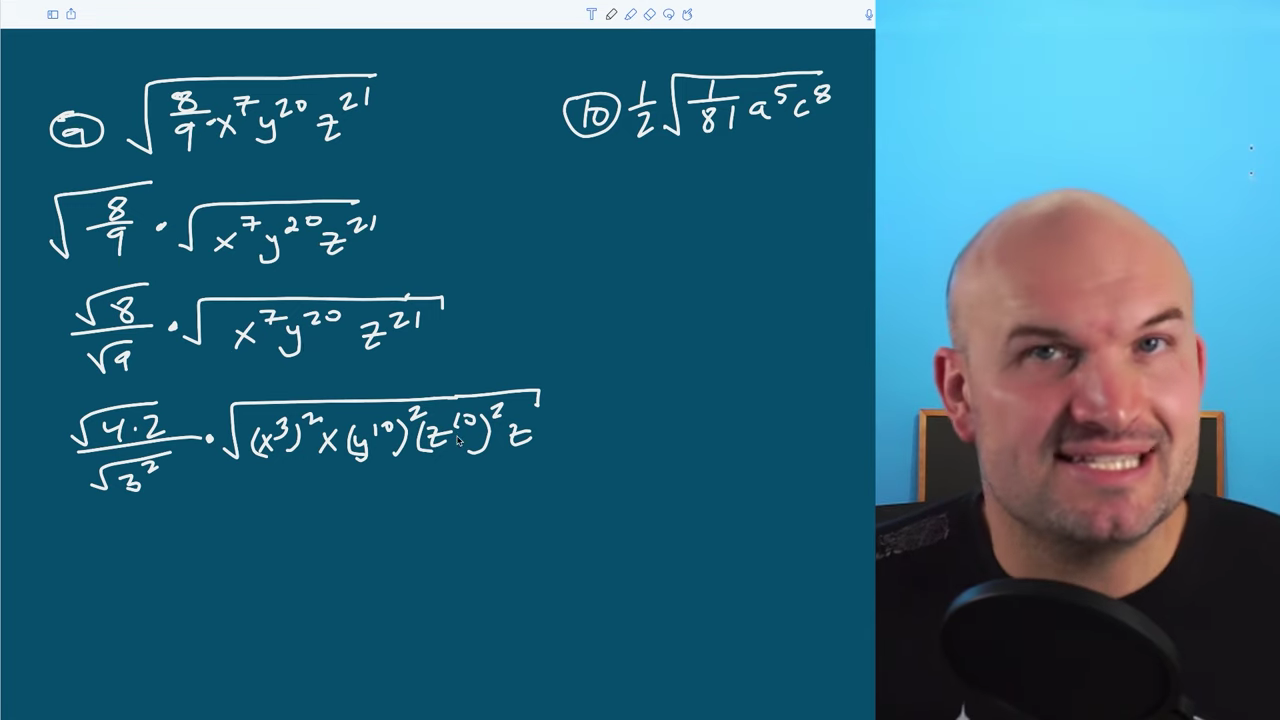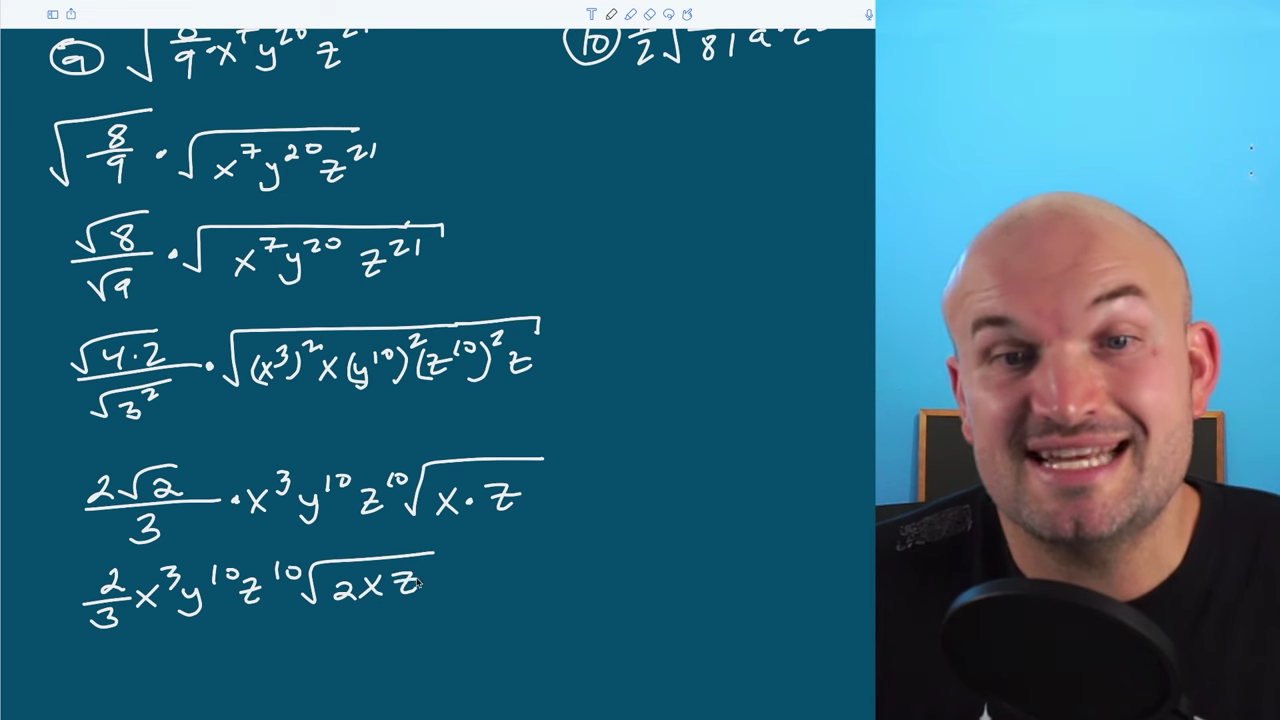Now, I’ll simplify by taking the square root of everything I can. I can take the square root of 4, leaving me with 2√2. The square root of 3² is 3. The square root of (x³)² is x³. I can take out the square root of y^10 and z^10. What’s left under my square root is xz. Now, I can simplify one more time and multiply the two radicals. Like we separated them, we can bring them back together and write it as a fraction; (2/3)x³y^10z^10√2xz.

## Example #10: 1/2sqrt(1/81a^5c^8)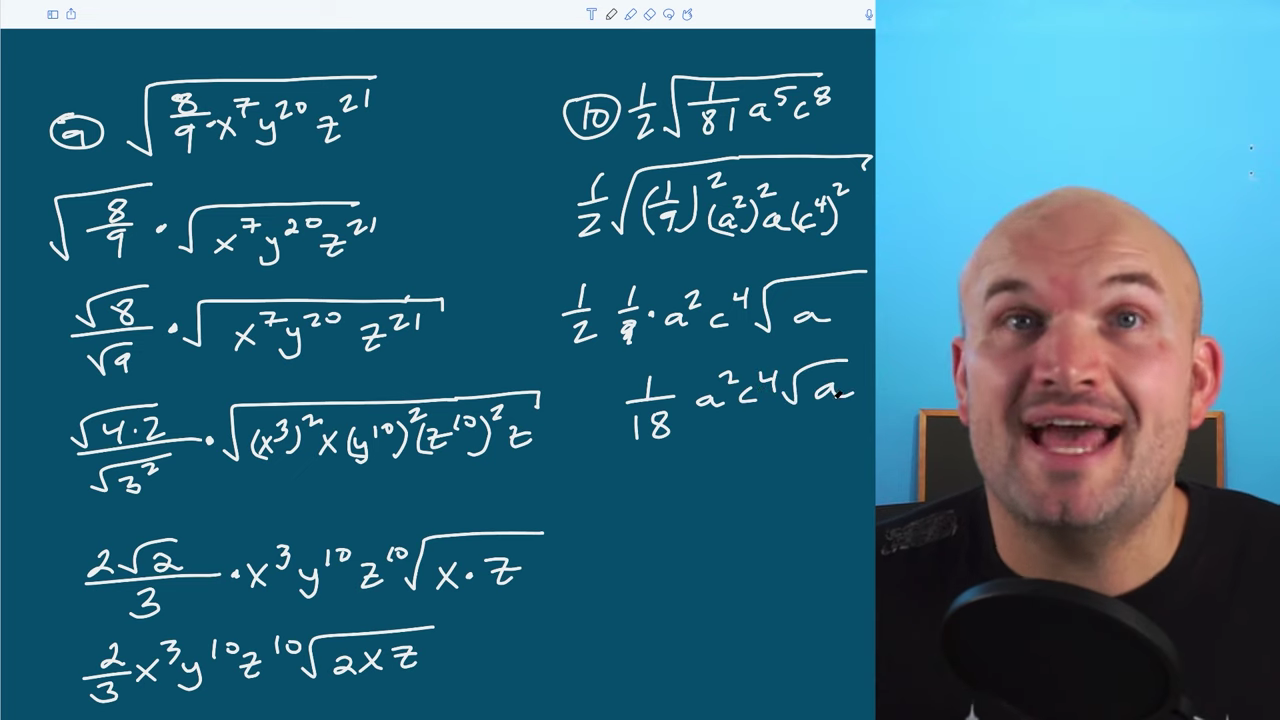In this example, you could just break up the radical, but the square root of 1 is 1, and the square root of 81 is 9, so I can rewrite this as √(1/9)² because (1/9)(1/9) = (1/81). I’ll rewrite a^4 and c^8 using the power and the product rule, and then I can take the square root of each of these terms squared. The 1/2 is my scalar multiple so I’ll leave it on the outside and get a final of (1/2)(1/9)⋅a²⋅c^4√a. I can simplify further and rewrite it as (1/18)a²c^4√a.

Those were ten examples of how to simplify the square root of a variable expression. I hope this was helpful but if you need more examples or you want to see a bigger variety, I’ll link some more examples below.